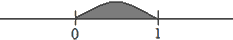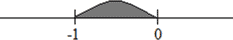# Proper Fraction

Let you have a fraction a ⁄ b, then how can you know if it's a proper fraction?

It's easy! Look at "a", then look at "b". What is greater?

If "b" is greater than "a" (b > a), it means this time you have a proper fraction.

##### Example:

3 ⁄ 8 (8 > 3)     11 ⁄ 57 (57 > 11)     1003 ⁄ 1020 (1020 > 1003)

### It's interesting!

Every fraction has its own place on the number scale.

Every positive proper fraction is always greater than "0" but less than "1".
(ex. 0 < 3 ⁄ 7 < 1)But every negative proper fraction is always between (-1) and "0".
(ex. -1 < -10 ⁄ 21 < 0)Is it a fractional number (0 ∈ Q ?)?

Sure, you may write it like this 0 = 0 ⁄ 19. So, you see "0" may be a proper fraction, too.

But we can't say the same about "1" and "-1".
Look, 1 = 4 ⁄ 4 = 7 ⁄ 7 = 8 ⁄ 8... and -1 = -5 ⁄ 5 = -11 ⁄ 11.

So, "1" and "-1" are fractional numbers (1 ∈ Q, -1 ∈ Q),
but you can't write "1" and "-1" as proper fractions.

4 ⁄ 4;     7 ⁄ 7;     11 ⁄ 11...     all they are improper fractions.

So, we can do addition, subtraction, multiplication, division, raising to a power, taking the logarithm with proper fractions. There are special rules for this.

### Multiplication

a ⁄ b × c ⁄ d = a × c ⁄ b × d; b ≠ 0, d ≠ 0

##### Example:

3 ⁄ 4 × 1 ⁄ 7 = 3 × 1 ⁄ 4 × 7 = 3 ⁄ 28 – proper fraction.

2 ⁄ 9 × 3 ⁄ 5 × 7 ⁄ 10 = 2 ×3 × 7 ⁄ 9 × 5 × 10 = 42 ⁄ 450 – proper fraction.

##### Notice!

You get always a proper fraction doing multiplication.

### Division

a ⁄ b ÷ c ⁄ d = a ⁄ b × d ⁄ c = a × d ⁄ b × c, c ≠ 0, d ≠ 0, b ≠ 0

##### Example:

3 ⁄ 4 ÷ 9 ⁄ 15 = 3 ⁄ 4 × 15 ⁄ 9 = 45 ⁄ 36 – not a proper fraction.

1 ⁄ 12 ÷ 1 ⁄ 7 = 1 ⁄ 12 × 7 ⁄ 1 = 7 ⁄ 12 – proper fraction.

##### Notice!

Doing division you may get not only a proper fraction.

### Raising to a power

(a ⁄ b)n = an ⁄ bn, b ≠ 0

(2 ⁄ 3)3 = 23 ⁄ 33 = 8 ⁄ 27 – proper fraction.

(1 ⁄ 4)2 = 12 ⁄ 42 = 1 ⁄ 16 – proper fraction.

##### Notice!

You get only a proper fraction doing raising to a power!

a ⁄ b ± x ⁄ y = (a × y ± x × b) ⁄ b × y, if b ≠ 0, y ≠ 0

##### Example:

3 ⁄ 4 + 2 ⁄ 5 = (3 × 5 + 2 × 4) ⁄ 4 × 5 = (15 + 8) ⁄ 20 = 23 ⁄ 20 – not a proper fraction.

3 ⁄ 4 - 2 ⁄ 5 = (3 × 5 - 2 × 4) ⁄ 4 × 5 = (15 - 8) ⁄ 20 = 7 ⁄ 20 – proper fraction.

##### Notice!

Doing addition and subtraction you can get not only a proper fraction.

So, isn't it true: "One head is good, but two are better"?

#### Note

- fraction slash

= - is equal to; equals

- is not equal to; does not equal

< - is less than

> - is greater than

× - multiplied by

÷ - divided by

± - plus or minus